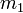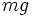# Gravitational force

## Definition

### In general

Gravitational force is a body force experienced between two bodies. The line of action of gravitational force is the line joining the centers of mass of the two bodies and the direction is inwward for both (i.e., the direction of gravitational force on one body is toward the center of mass of the other). The magnitude of gravitational force is:$\frac{Gm_1m_2}{R^2}$

where$G$ is the gravitational constant,$m_1$ and$m_2$ are the masses of the bodies, and$R$ is the distance between their centers of mass.

### For a body near the surface of the earth

For a body of small size near the surface of the earth, the gravitational force acts in a downward direction on the body and its magnitude is$mg$, where$g$ is the acceleration due to gravity near the surface of the earth.

The same principle applies for bodies near the surface of planets, satellites, stars, and other large roughly spherical astronomical bodies, though the value of acceleration due to gravity may differ.